On this page:     Paired fields     One to many     One (concatenated) to many

The recipes on this page involve cross-checking between two or more fields. They are useful not only for checking field content (and for finding pseudo-duplicates), but also for checking field format.

TSV This marker means that the recipe only works with tab-separated data tables.

## Paired fields

The "fldpair" function checks whether paired fields are both filled, neither filled or only one filled in individual records. An example of paired fields is a latitude field and a longitude field. It makes little sense to have a latitude without a longitude or vice-versa, and a data table with these fields should have either both filled or neither filled.

"fldpair" takes 3 arguments: filename, number of first field of pair, number of second field of pair. Field names are taken from the header line of the table. The screenshot below shows "fldpair" at work on 3 pairs of fields in the file "prep".

Tally paired fields    TSV

fldpair() { awk -F"\t" -v one="\$2" -v two="\$3" 'NR==1 {x=\$one; y=\$two; next} (\$one!="") && (\$two!="") {both++} (\$one!="") && (\$two=="") {oneonly++} (\$one=="") && (\$two!="") {twoonly++} (\$one=="") && (\$two=="") {neither++} END {print "neither\t"neither"\n"x" only\t"oneonly"\n"y" only\t"twoonly"\nboth\t"both}' "\$1" | sed 's/\t\$/\t0/' | column -t -s \$'\t'; }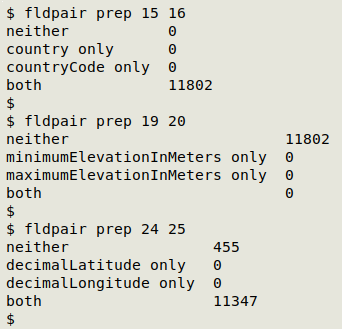Tally triplet fields    TSV

This is the 3-field version of "fldpair", called "fldtriplet":

fldtriplet() {
awk -F"\t" -v one="\$2" -v two="\$3" -v three="\$4" \
'NR==1 {x=\$one; y=\$two; z=\$three; next} \
(\$one != "") && (\$two != "") && (\$three != "") {allthree++} \
(\$one != "") && (\$two == "") && (\$three == "") {oneonly++} \
(\$one == "") && (\$two != "") && (\$three == "") {twoonly++} \
(\$one == "") && (\$two == "") && (\$three != "") {threeonly++} \
(\$one != "") && (\$two != "") && (\$three == "") {onetwoonly++} \
(\$one == "") && (\$two != "") && (\$three != "") {twothreeonly++} \
(\$one != "") && (\$two == "") && (\$three != "") {onethreeonly++} \
(\$one == "") && (\$two == "") && (\$three == "") {none++} \
END {print \
"none\t" none "\n" \
x " only\t" oneonly "\n" \
y " only\t" twoonly "\n" \
z " only\t"threeonly "\n" \
x " + " y " only\t" onetwoonly "\n" \
x " + " z " only\t" onethreeonly "\n" \
y " + " z " only\t" twothreeonly "\nall three\t" allthree}' "\$1" \
| sed 's/\t\$/\t0/' | column -t -s \$'\t'
}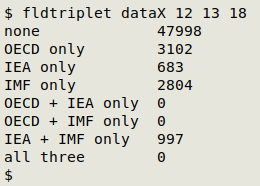## One to many

The "one2many" function is used for paired fields in which each unique, normalised value in the first field should have one and only one value paired with it in the second field. Like "fldpair", "one2many" takes 3 arguments: filename, number of first field of pair, number of second field of pair. As well as the unique first-field value and multiple second-field values, "one to many" prints the number of records for each case, and separates similar sets of data items with a blank line.

Check for one to many in paired fields    TSV

one2many() { awk -F"\t" -v one="\$2" -v many="\$3" '\$one != "" {a[\$one]++; b[\$one][\$many]++} END {for (i in b) {for (j in b[i]) {if (a[i]>b[i][j]) print b[i][j] FS i FS j} print RS}}' "\$1" | cat -s; }

In the example below, the first field (37) in file "tpm" contains county names and the second field (36) contains province names. There should be only one province entry for each county, but "one2many" has found disagreements.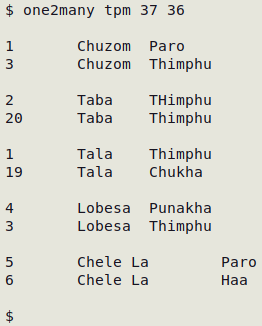The following variation on the recipe adds a header with fieldnames to the output, and replaces tabs in the output with [space][bar][space]:

one2manyh() { awk -F"\t" -v one="\$2" -v many="\$3" 'NR==1 {print "No. of records" FS \$one FS \$many; next} \$one != "" {a[\$one]++; b[\$one][\$many]++} END {for (i in b) {for (j in b[i]) {if (a[i]>b[i][j]) print b[i][j] FS i FS j} print RS}}' "\$1" | cat -s | sed 's/\t/ | /g'; }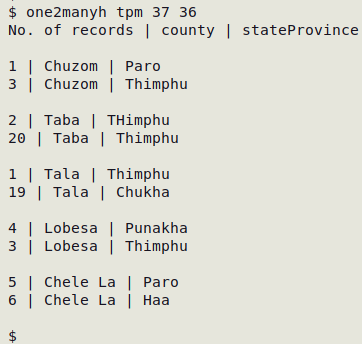For one way to fix large numbers of "one2many" errors, see here.

## One (concatenated) to many

This function extends "one2many" so that the first "field" is actually two fields with unique, normalised values, concatenated with a comma. In my data auditing, I often check tables that have a verbal location field, a latitude (or northing) field and a longitude (or easting) field. It sometimes happens that the same location is written in several different ways in the verbal field, like "3 km W of Route 6 and Route 3 junction" and "3km west jcn Routes 6 and 3", but the same way in the coordinate fields. To find these verbal location variants I use "one2manyLL", but the same function can be used for similar, non-geographic fields that are logically related.

Check for one to many in paired fields where the first is two concatenated fields    TSV

one2manyLL() { awk -F"\t" -v lat="\$2" -v lon="\$3" -v verbal="\$4" '\$lat !="" {a[\$lat","\$lon]++; b[\$lat","\$lon][\$verbal]++} END {for (i in b) {for (j in b[i]) {if (a[i]>b[i][j]) print b[i][j] FS i FS j} print RS}}' "\$1" | cat -s; }

In the table "afmo", latitude is field 27, longitude is field 28 and location name is field 24. The output below only shows the results for the first 3 cases where the same latitude/longitude combination had different location strings.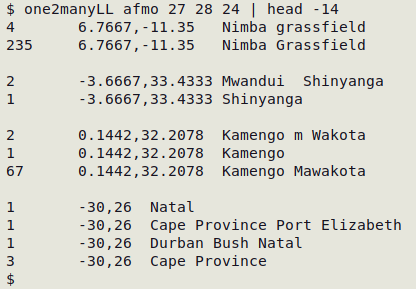As with "one2manyh", the following variation adds a header with fieldnames and replaces tabs in the output with [space][bar][space]:

one2manyLLh() { awk -F"\t" -v lat="\$2" -v lon="\$3" -v verbal="\$4" 'NR==1 {print "No. of records" FS \$lat","\$lon FS \$verbal; next} \$lat != "" {a[\$lat","\$lon]++; b[\$lat","\$lon][\$verbal]++} END {for (i in b) {for (j in b[i]) {if (a[i]>b[i][j]) print b[i][j] FS i FS j} print RS}}' "\$1" | cat -s | sed 's/\t/ | /g'; }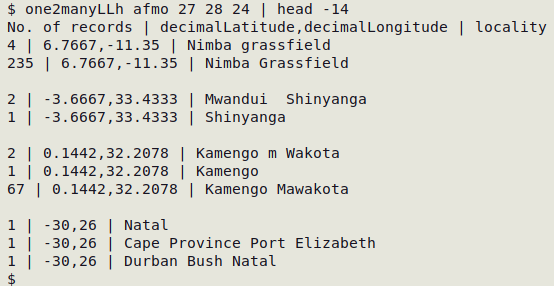For one way to fix large numbers of "one2manyLL" errors, see here.